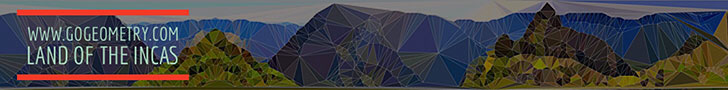Geometry Problems 151-160: Triangle, Quadrilateral, Area, Circle, Similarity, Center# Geometry Problems 151 - 160

Proposed Problem 151. Quadrilateral, Area, Trisection of Sides.

Proposed Problem 152. Circumscribed Quadrilateral, Diagonal, Chord, Proportion.

Proposed Problem 153. Circumscribed Quadrilateral, Diagonals Concurrent with Chords.

Proposed Problem 154. Triangle, Inradius, Circumradius, Chord.

Proposed Problem 155. Euler's Theorem: Distance between the Incenter to the Circumcenter.

Proposed Problem 156. Triangle, Circumradius, Exradius, Chord, Secant line.

Proposed Problem 157. Distance from the Circumcenter to the Excenter.

Proposed Problem 158. Relation between the Circumradius, Inradius and Exradii of a triangle.

Proposed Problem 159. Distances from the Circumcenter to the Incenter and the Excenters.

Proposed Problem 160. Triangle, Incircle, Incenter, Circumcircle, Circumcenter, Inradius.

 Home | Sitemap | Search | Geometry | Problems | Visual Index | 10 Problems | Email | Post a comment | By Antonio Gutierrez